## Assume a substance X has a body centered cubic lattice. The edge length is 379.0 pm. The atomic mass of X is 195.0 amu What is the density o

Question

Assume a substance X has a body centered cubic lattice. The edge length is 379.0 pm. The atomic mass of X is 195.0 amu What is the density of Xin g/cc

in progress 0
6 months 2021-07-31T03:26:26+00:00 1 Answers 3 views 0

## Answers ( )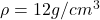Explanation:

From the question we are told that:

Length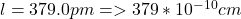Atomic mass of X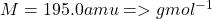Where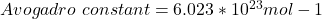Let

The Number of units in BCC unit cell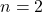Generally the equation for Density is mathematically given by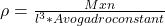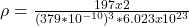Therefore he density of Xin g/cc